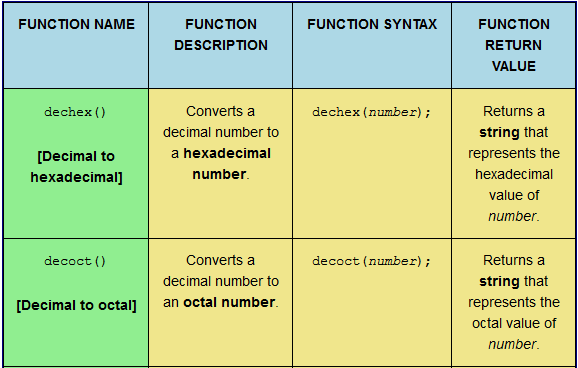# PHP Mathematical Function Quiz: `round()`; `sin()`; `sinh()`; `sqrt()`; `srand()`; `tan()`; and `tanh()`.This web page provides a reference table and a subsequent quiz to aid learning seven PHP mathematical functions: `round()`; `sin()`; `sinh()`; `sqrt()`; `srand()`; `tan()`; and `tanh()`. The quiz involves matching the function description, function syntax, and function return value to the correct function name. Different table formats are available in order to cater for users on a desktop computer, a laptop, or a mobile phone.

Tagged with: , , , ,
Posted in PHP Maths Quizzes

# PHP Mathematical Function Quiz: `mt_rand()`; `mt_srand()`; `octdec()`; `pi()`; `pow()`; `rad2deg()`; and `rand()`.This web page provides a reference table and a subsequent quiz to aid learning seven PHP mathematical functions: `mt_rand()`; `mt_srand()`; `octdec()`; `pi()`; `pow()`; `rad2deg()`; and `rand()`. The quiz involves matching the function description, function syntax, and function return value to the correct function name. Different table formats are available in order to cater for users on a desktop computer, a laptop, or a mobile phone.

Tagged with: , , , ,
Posted in PHP Maths Quizzes

# PHP Mathematical Function Quiz: `lcg_value()`; `log10()`; `log1p()`; `log()`; `max()`; `min()`; and `mt_getrandmax()`.This web page provides a reference table and a subsequent quiz to aid learning seven PHP mathematical functions: `lcg_value()`; `log10()`; `log1p()`; `log()`; `max()`; `min()`; and `mt_getrandmax()`. The quiz involves matching the function description, function syntax, and function return value to the correct function name. Different table formats are available in order to cater for users on a desktop computer, a laptop, or a mobile phone.

Tagged with: , , , ,
Posted in PHP Maths Quizzes

# PHP Mathematical Function Quiz: `getrandmax()`; `hexdec()`; `hypot()`; `intdiv()`; `is_finite()`; `is_infinite()`; and `is_nan()`.This web page provides a reference table and a subsequent quiz to aid learning seven PHP mathematical functions: `getrandmax()`; `hexdec()`; `hypot()`; `intdiv()`; `is_finite()`; `is_infinite()`; and `is_nan()`. The quiz involves matching the function description, function syntax, and function return value to the correct function name. Different table formats are available in order to cater for users on a desktop computer, a laptop, or a mobile phone.

Tagged with: , , , ,
Posted in PHP Maths Quizzes

# PHP Mathematical Function Quiz: `dechex()`; `decoct()`; `deg2rad()`; `exp()`; `expm1()`; `floor()`; and `fmod()`.This web page provides a reference table and a subsequent quiz to aid learning seven PHP mathematical functions: `dechex()`; `decoct()`; `deg2rad()`; `exp()`; `expm1()`; `floor()`; and `fmod()`. The quiz involves matching the function description, function syntax, and function return value to the correct function name. Different table formats are available in order to cater for users on a desktop computer, a laptop, or a mobile phone.

Tagged with: , , , ,
Posted in PHP Maths Quizzes

# PHP Mathematical Function Quiz: `atanh()`; `base_convert()`; `bindec()`; `ceil()`; `cos()`; `cosh()`; and `decbin()`.This web page provides a reference table and a subsequent quiz to aid learning seven PHP mathematical functions: `atanh()`; `base_convert()`; `bindec()`; `ceil()`; `cos()`; `cosh()`; and `decbin()`. The quiz involves matching the function description, function syntax, and function return value to the correct function name. Different table formats are available in order to cater for users on a desktop computer, a laptop, or a mobile phone.

Tagged with: , , , ,
Posted in PHP Maths Quizzes

# PHP Mathematical Function Quiz: `abs()`; `acos()`; `acosh()`; `asin()`; `asinh()`; `atan()`; and `atan2()`.This web page provides a reference table and a subsequent quiz to aid learning seven PHP mathematical functions: `abs()`; `acos()`; `acosh()`; `asin()`; `asinh()`; `atan()`; and `atan2()`. The quiz involves matching the function description, function syntax, and function return value to the correct function name. Different table formats are available in order to cater for users on a desktop computer, a laptop, or a mobile phone.

Tagged with: , , ,
Posted in PHP Maths Quizzes

# PHP QUIZ: All Mathematical FunctionsThis web page provides a reference table and a subsequent quiz to aid learning forty-nine PHP mathematical functions. The quiz involves matching the function description, function syntax, and function return value to the correct function name. Different table formats are available in order to cater for users on a desktop computer, a laptop, or a mobile phone.

Tagged with: , , , ,
Posted in PHP Maths Quizzes